## When to Use OptoScript

Let's take a look at some examples of tasks that would be easier to do in OptoScript.

• Math expressions
• String handling
• Complex loops
• Case statements
• Conditions
• Combining all of the above

Math Expressions

Math expressions are simpler in OptoScript, and many of them are built right into the language. For example, you don't need PAC Control commands called Add or Multiply.

OptoScript has no limitations on the number of parentheses used in math expressions. Here’s an example of a mathematical expression in OptoScript:

integer1 = (integer2 + 2) * (float1 / (float2 - 2) - 3);

To do the same using standard PAC Control commands, you'd need to create at least two intermediate variables and use five instructions, as in this example.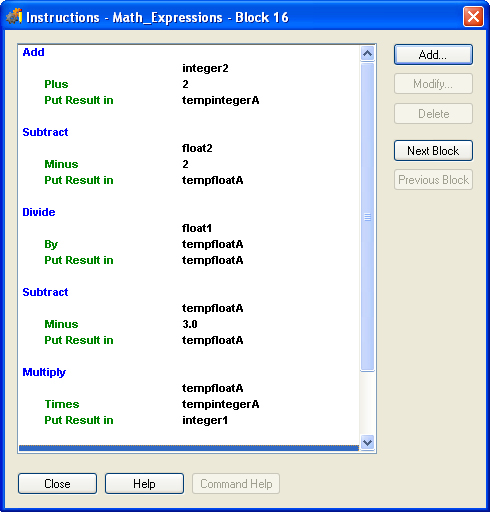String Handling

If your strategy sends and receives serial data, you'll want to use OptoScript code. In standard PAC Control command blocks, forming and parsing (decoding) serial data can take several blocks. In OptoScript, string handling is easier.

Here's a flowchart that sends the string request, “What type of plane?”, and parses the response.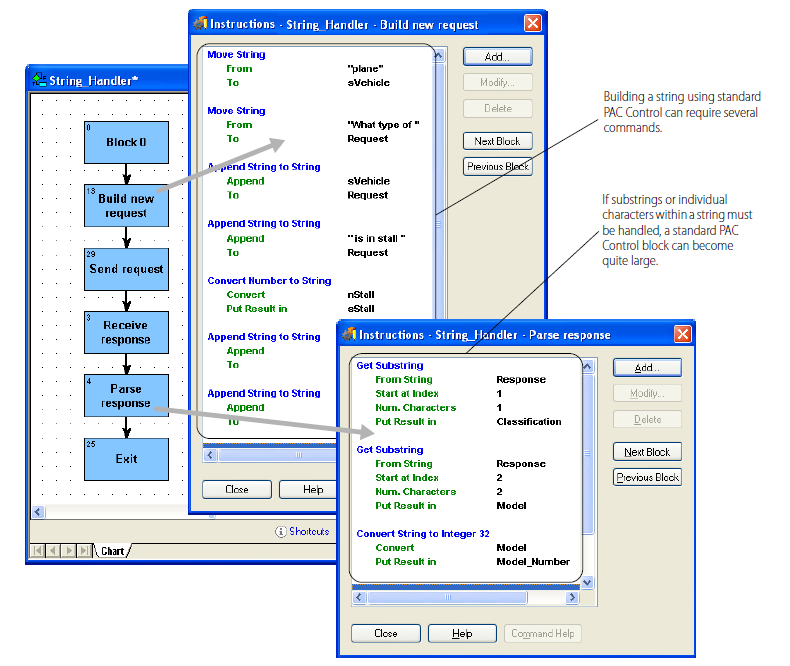Compare these blocks and instructions with the ones done in OptoScript.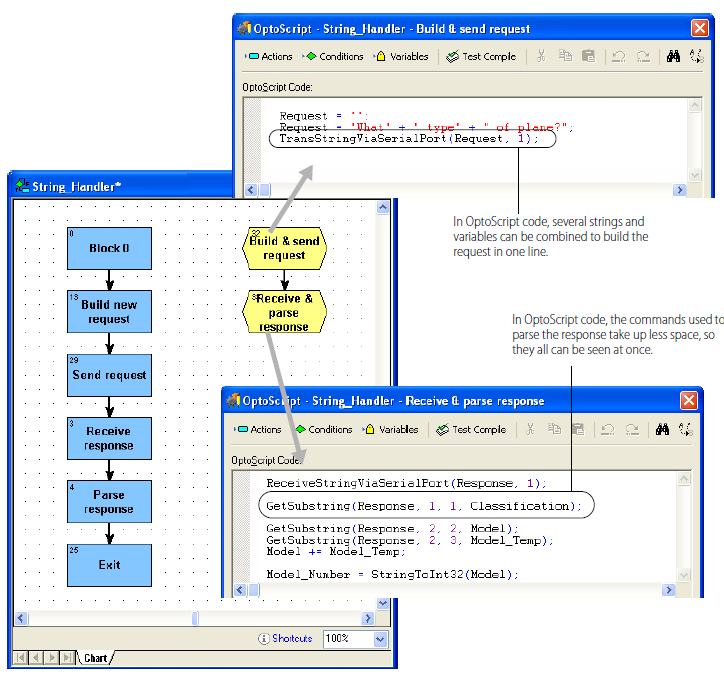Complex Loops

Strategies that use complex loops—for example, to repeat an operation while a condition remains true—are easier to create and take up less space in a flowchart when done in OptoScript. While loops, repeat loops, and for loops are all available.

• While loops: Repeats a process while a test is true. The test is done at the beginning of the process.
• Repeat loops: Repeats a process until a test is false The test comes at the end of the process. This type of loop executes the programming code at least once.
• For loops: Repeats a process for a specified number of times.

While Loops

Below is an example of a while loop as it would appear in standard flowchart commands, contrasted with the way it could be handled in an OptoScript block.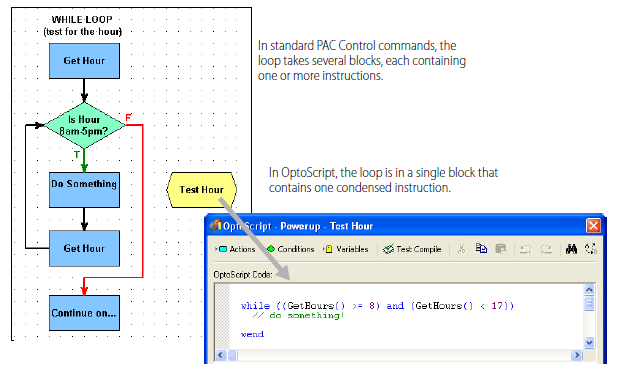Case Statements

Case or switch statements create multiple decision points. They can also be easier to do using OptoScript. Here is an example of a case statement: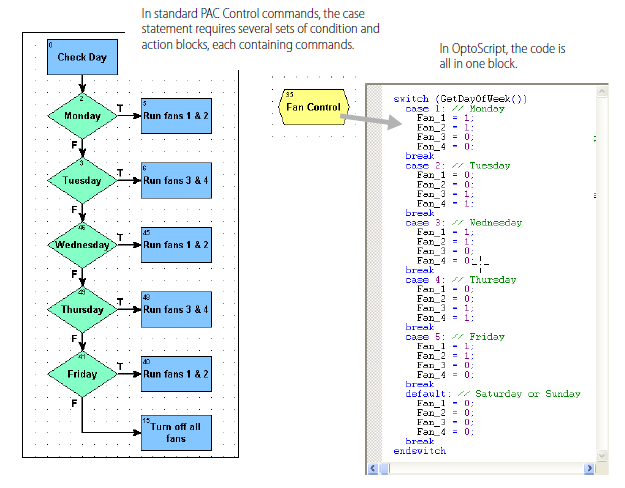Conditions

Like loops and case statements, conditions can be simpler when done in OptoScript code. If/then, if/then/else, and if/then/elseif statements can all be mixed and nested as needed. Here’s an example of a simple if/then/else statement in standard PAC Control commands and in OptoScript: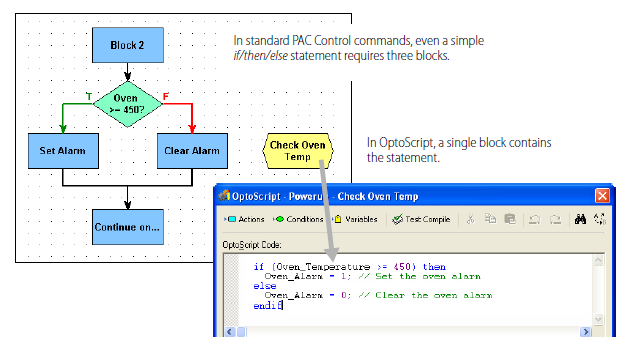OptoScript is even more useful for more complex conditions, such as the following: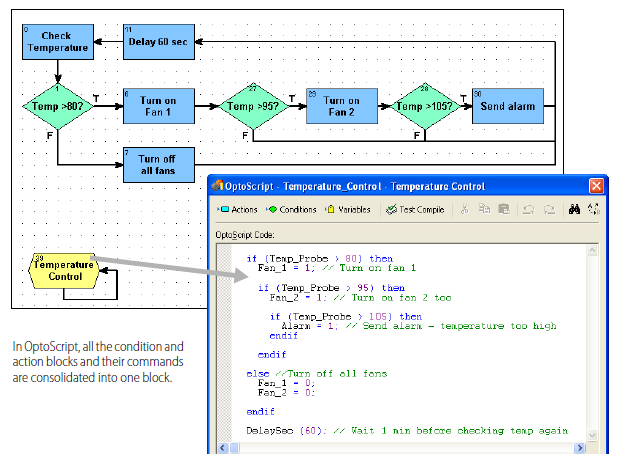Combining Expressions, Operators, and Conditions

The real power of OptoScript can be seen in complex operations.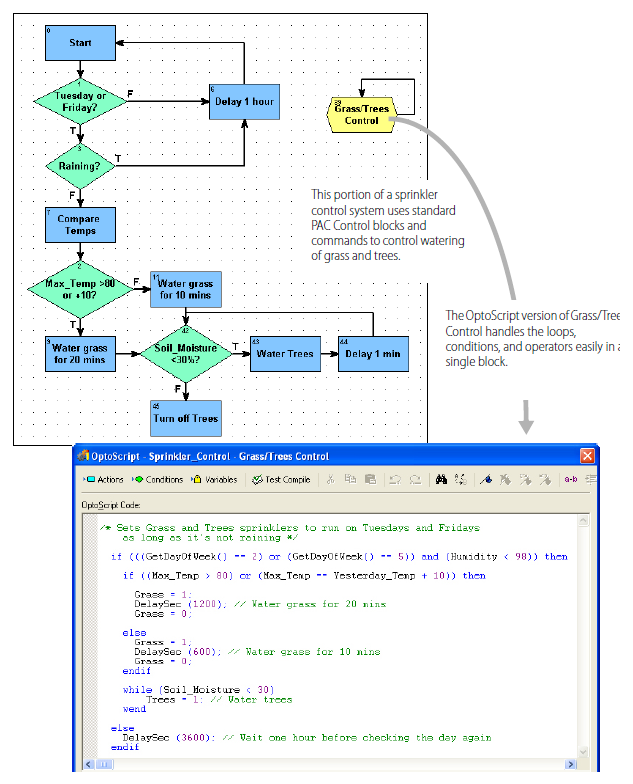In summary, the more complex the combination of math expressions, logical and comparison operators, loops, and conditions, the more convenient it is to use OptoScript code rather than standard blocks and commands.

#### Summary

This lesson takes a look at some examples of tasks that would be easier to do in OptoScript than in PAC Control blocks.

• Math expressions
• String handling
• Complex loops
• Case statements
• Conditions
• Combining all of the above